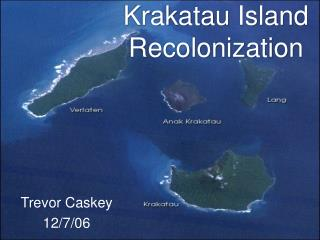DownloadDownload PresentationKrakatau Island Recolonization

# Krakatau Island Recolonization

Télécharger la présentation## Krakatau Island Recolonization

- - - - - - - - - - - - - - - - - - - - - - - - - - - E N D - - - - - - - - - - - - - - - - - - - - - - - - - - -
##### Presentation Transcript

1. Krakatau Island Recolonization Trevor Caskey 12/7/06

2. History • Krakatau island lies in the Sundra Straight between Java and Sumatra in Indonesia.

3. 1883 Eruption • Krakatau erupted on August 27, 1883. • One of the largest eruption ever recorded. • As powerful as 21,547 atom bombs • Destroyed 75% of island and sent a tsunami towards Java and Sumatra killing 36+ thousand people. • No life remained on the islands.

4. Recolonization of the Islands • The idea of studying and modeling plant dispersal on bare islands was brought up my K.W. Dammerman in 1948 and made into an actual field of study (Biogeography) by MacArthur and Wilson in the there book “ The Theory of Island Biogeography”(1963). • Scientists kept surveys of floral and faunal species on Krakatau island from 1883 thru the 1930’s which were the basis for Dammerman, and MacArthur and Wilson’s work. • From examining the data of these studies, animals (esp. birds) were the cause of approx. 25% of the plant species.

5. PTOTAL = Total number of plant species PPLANT = Number of plant species NOT introduced by birds PBIRDS = Number of plant species introduced by birds B = Number of bird species on the island

6. Metapopulation Modeling • Basic Levin’s Model: dP/dt = cP(1-P)-eP P= population c = colonization rate e = extinction rate Mathematically this is the logistic model, which is used when there is limited environment for growth. r = growth rate P = population K = Carrying Capacity

7. This project is aimed at showing even though the carrying capacity KPP of the island is limited by the area of the island, KPP can be exceeded due to bird immigration. • The Model: r1 = Colonization rate Pp r2 = Unknown factor to be found r3 = Colonization rate B KPp = Carrying Capacity of Pp KB = Carrying Capacity B

8. From linear regression of the data, r1 and r3 were found. r1 =0.267186 r3 = 1.582925 From looking at the data, KPp and KB are assumed to be: KPp = 203 KB = 27

9. The solutions of the equations are: To find r2: Set P(t) to 271, B0=27, Pp0=203, t=51(1934) (from the data table) r2 = 0.110566

10. Given our finished equations, we can now check them to see if they match the data. • At t=3 (1886) B(3) = 0, P(3) =